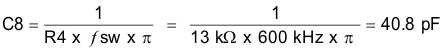JAJSBY0G August   2012  – June 2018

PRODUCTION DATA.

1. 特長
2. アプリケーション
3. 概要
1.     Device Images
4. 改訂履歴
5. Pin Configuration and Functions
6. Specifications
7. Detailed Description
1. 7.1 Overview
2. 7.2 Functional Block Diagram
3. 7.3 Feature Description
4. 7.4 Device Functional Modes
8. Application and Implementation
1. 8.1 Application Information
2. 8.2 Typical Application
1. 8.2.1 Design Requirements
2. 8.2.2 Detailed Design Procedure
3. 8.2.3 Application Curves
9. Power Supply Recommendations
10. 10Layout
1. 10.1 Layout Guidelines
2. 10.2 Layout Example
11. 11デバイスおよびドキュメントのサポート
12. 12メカニカル、パッケージ、および注文情報

• DDA|8
• DDA|8

#### 8.2.2.11 Compensation

There are several methods to design compensation for DC/DC regulators. The method presented here is easy to calculate and ignores the effects of the slope compensation that is internal to the device. Since the slope compensation is ignored, the actual crossover frequency will be lower than the crossover frequency used in the calculations. This method assumes the crossover frequency is between the modulator pole and the ESR zero and the ESR zero is at least 10 times greater the modulator pole.

To get started, the modulator pole, ƒp(mod), and the ESR zero, ƒz1 must be calculated using Equation 44 and Equation 45. For COUT, use a derated value of 58.3 μF. Use equations Equation 46 and Equation 47 to estimate a starting point for the crossover frequency, ƒco. For the example design, ƒp(mod) is 1912 Hz and ƒz(mod) is 1092 kHz. Equation 45 is the geometric mean of the modulator pole and the ESR zero and Equation 47 is the mean of modulator pole and the switching frequency. Equation 46 yields 45.7 kHz and Equation 47 gives 23.9 kHz. Use the lower value of Equation 46 or Equation 47 for an initial crossover frequency. For this example, the target ƒco is 23.9 kHz.

Next, the compensation components are calculated. A resistor in series with a capacitor is used to create a compensating zero. A capacitor in parallel to these two components forms the compensating pole.

Equation 44.Equation 45.Equation 46.Equation 47.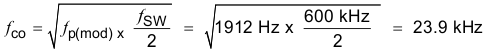To determine the compensation resistor, R4, use Equation 48. Assume the power stage transconductance, gmps, is 12 A/V. The output voltage, VO, reference voltage, VREF, and amplifier transconductance, gmea, are 5 V, 0.8 V and 350 μA/V, respectively. R4 is calculated to be 13 kΩ which is a standard value. Use Equation 49 to set the compensation zero to the modulator pole frequency. Equation 49 yields 6404 pF for compensating capacitor C5. 6800 pF is used for this design.

Equation 48.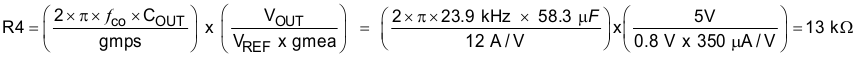Equation 49.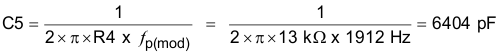A compensation pole can be implemented if desired by adding capacitor C8 in parallel with the series combination of R4 and C5. Use the larger value calculated from Equation 50 and Equation 51 for C8 to set the compensation pole. The selected value of C8 is 39 pF for this design example.

Equation 50.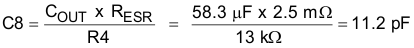Equation 51.